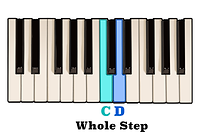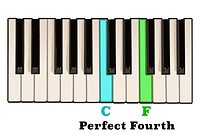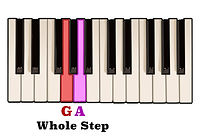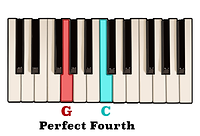top of page

## Interval Identification | Perfect Fourths (4 of 10)

An interval of a Perfect fourth is labelled as "perfect" because the vibrational frequency of the two notes is in greater harmony with each other than other intervals such as seconds, thirds, sixths, and sevenths.

For example, the ratio of the vibrational frequency of an interval of a Perfect fourth is 4/3.

This means that an interval of a Perfect fourth above any given note vibrates at a frequency of one and a third more than the original note.

In other words, if a note vibrates at a frequency of approximately three hundred hertz, or three hundred cycles per second, then an interval of a Perfect fourth above that note would vibrate at a frequency of approximately four hundred cycles per second.

Put more simply, an interval of a Perfect fourth occurs when two notes are separated by the distance of five consecutive half steps.

If two half steps equals one whole step then five half steps is comparatively the same as two whole steps and one half step. Let's use this information to figure out what an interval of a Perfect fourth above the note C is.Starting on the note C and going up one whole step takes us to the note D.Another whole step above D is the note E.And finally, a half step above E is the note F.As you can see, an interval of a Perfect fourth above the note C is the note F.

In the previous video discussing intervals of a third, we saw how both an interval of a Major third and an interval of a minor third above any given note can also be found as being the third scale degree of that note's corresponding Major or minor scale.

This same method may be applied to intervals of a fourth as well. However, an interval of a Perfect fourth above any given note will always be found on the fourth scale degree of both a Major and minor scale.

We just learned that because they are separated by five consecutive half steps, the distance between the notes C and F is an interval of a Perfect fourth. If we take a look at both a C Major scale and a C minor scale, we can see that the fourth note in both scales is in fact F natural.Although it can be a convenient method for figuring out different intervals, it is important to remember that the true definition of an interval is not necessarily its position in the scale in relation to the root note. Rather, an interval is more accurately defined as the amount of steps separating any two pitches, or notes.

We have already seen that in both a C Major and C minor scale an interval of a Perfect fourth above the note C is the note F. However, this is not the only Perfect fourth interval which occurs within those scales. The interval between the fifth scale degree, G, and the octave of the first scale degree, C, meaning the root note found after the seventh scale degree, is also an interval of an ascending Perfect fourth.Let's double check by counting the amount of steps separating the note G and the first C above it.A whole step above the note G is the note A.Another whole step above A is the note B.And finally, a half step above B is the note C.An interval of a perfect fourth above G is the note C.

As its name would suggest, an interval of a fourth is any interval which spans four positions on the staff. When reading intervals of a Perfect fourth on the staff, you will notice that the two notes always go either from a line to a space with two staff positions between them,or from a space to a line, also with two staff positions between them.For example, if the lower note of the interval is on a space, then the top note will have skipped the next line and space above it and be placed on the following line. Likewise, if the lower note is on a line, then the top note will skip the next space and line and be placed on the following space. Memorizing this shape on the staff will make it easier to quickly recognize intervals of a Perfect fourth when reading and writing music.

bottom of page